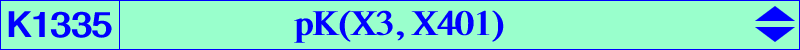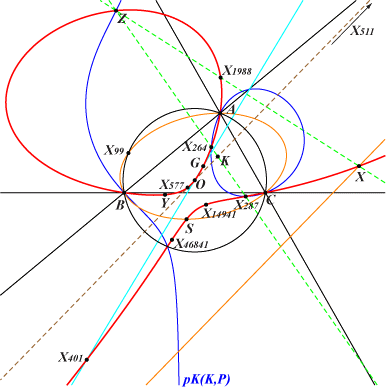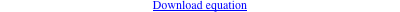too complicated to be written here. Click on the link to download a text file.X(2), X(3), X(264), X(287), X(401), X(511), X(577), X(1988), X(5374), X(14941), X(46841), X(54976) X(57008) → X(57012), X(57253), X(57254), X(57273), X(57274) infinite points of the Steiner ellipses see points below Geometric properties :K1335 is the SS{X3} transform of K323 and the SS{X216} transform of K623. See Table 70 and also CL041 (green lines in the second table). K1335 meets the Steiner ellipse again at S = X(54976), on the line X(99), X(1092). S is the SS{X3} transform of X(3226). K1335 meets the circumcircle again at the same points as pK(X6, P), with P = X(57275), on the lines {X22, X69}, {X99, X2706}, {X147, X325}, {X154, X183}. P is the reflection of X(385) in X(1971). K1335 meets its real asymptote at X, on the lines {X511, X1987}, {X1988, X3167}. Y = X(57008) = X3 ÷ X1998 on the lines {X4, X69}, {X99, X1092} and Z = X(401)-Ceva conjugate of X(3) = X(57012) on the lines {X3, X1625}, {X6, X264}, {X155, X2782} are the SS{X3} transforms of X(3212) and X(24578) respectively. The third point of K1335 on the line {X99, X1092} is the SS{X3} transform X(57011) of X(8301). It lies on {X2, X98}, {X115, X578}. X(57009), X(57010), X(57254), X(57273), X(57274) are the SS{X3} transforms of X(52160), X(17731), X(33888), X(9278), X(2669) respectively.